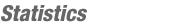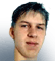JOINMatch Editorial
SRM 144
Wednesday, April 30, 2003

Match summary

ZorbaTHut was dominant in SRM 144, outscoring second place SnapDragon by almost 150 points - without the aid of any challenges. His strong performance gave him 116 points, and moved him up to 6th overall. SnapDragon came in second as the only other coder with 3 correct submissions. Overall, the problems were a little more difficult than usual, and were scored as such. In division 2, bladerunner, a new comer, led the pack by an equally large margin, beating second place jorend by almost 200 points. Congrats to ZorbaTHut and bladerunner.

# The Problems

TimeUsed as: Division-II, Level 1:
 Value 200 Submission Rate 234 / 252 (92.86%) Success Rate 212 / 234 (90.60%) High Score haro for 199.20 points

#### Implementation

This problem could be solved pretty simply with a couple of division and modulus operators. The important thing here was to understand the % operator which gives you the remainder from a division. Thus, you could find <H>, <M> and <S> easily as follow:

```int h = seconds/3600;
int m = (seconds%3600)/60;
int s = seconds%60;
```

Binary CodeUsed as: Division-II, Level 2:
 Value 550 Submission Rate 141 / 252 (55.95%) Success Rate 33 / 141 (23.40%) High Score bladerunner for 440.91 points
Used as: Division-I, Level 1:
 Value 300 Submission Rate 96 / 104 (92.31%) Success Rate 66 / 96 (68.75%) High Score SnapDragon for 289.24 points

#### Implementation

This problem was a little bit tricky at first glance. However, the examples in the problem statement gave you a good idea of how to do it. Once you make an assumption about the value of the first bit, you can then determine the value of the next bit, and so one until you get to the last bit. If, while you are going along, you find that you would have to set a bit to a number other than 0 or 1 to make the sum work out, then the assumption you made at the beginning has caused some sort of inconsistency, and leads to the answer "NONE". One tricky thing is that, when you get to the last bit, you have to look ahead and see what the next bit would have to be for the given encoding to occur. Since the last bit is off the end of the string, it has to be 0. If the encoding requires it to be anything else, you again have an inconsistency. Most people who failed seemed to make mistakes either with the single digit case, or in checking the end digit.

PowerOutageUsed as: Division-II, Level 3:
 Value 1100 Submission Rate 24 / 252 (9.52%) Success Rate 9 / 24 (37.50%) High Score bladerunner for 872.75 points

#### Implementation

In this problem we are given series of tunnels, which can be represented as a rooted tree, and are asked to find the total path length required to visit every node at least once, starting from node 0. The way to solve this problem is to think about how you would do it if you had to. First, you would go down one tunnel leading away from tunnel 0, and then go down all of the tunnels leading away from that one. In other words, you should completely explore a subtree before returning to the root to explore another subtree. If you think about this a little, and draw some trees, you see that every edge gets traversed exactly once. However, at the end, you don't have to go back to the root. Once you have visited all of the nodes, you are done. So, it makes sense to end your search at the node that is farthest away from the root. That way, you have to traverse all of the edges from the root to that node one less time. Thus, the total distance turns out to be (2 * sum of all edge weights) - (length of the longest path starting at the root).

LotteryUsed as: Division-I, Level 2:
 Value 550 Submission Rate 52 / 104 (50.00%) Success Rate 45 / 52 (86.54%) High Score ZorbaTHut for 482.98 points

#### Implementation

There are a couple of different ways to solve this. One of them involves dynamic programming, and the other involves combinatorics. I will describe the combinatoric approach, which is simpler to code, if you can come up with it.

First, it is clear that most of the problem involves counting the number of possible ways to fill in each lottery ticket. After that it's just sorting. There are 4 cases to consider here.

1. The easiest is when both unique and sorted are false. There are then choices options for blanks spaces, for a total of choicesblanks total tickets.
2. The next easiest case is when they only have to be unique. There are choose(choices,blanks) ways to choose blanks numbers to fill in, and fact(blanks) ways to order each set. So, there are choose(choices,blanks) * fact(blanks) total ways to fill in a ticket that must be unique. For those of you not familiar with it, the function choose(n,m) is a binomial coefficient and is given by fact(n)/fact(m)/fact(n-M) and gives the number of ways to choose m things out of n total (read more at MathWorld). fact(blanks) then gives the number of ways to order the chosen numbers.
3. Perhaps equally easy is when they must be both unique and sorted. Similarly, there are choose(choices,blanks) ways to pick your numbers, but now there is only one way to fill them in - sorted. Thus the number of ways to fill in the ticket when sorted and unique are both true is just choose(choices,blanks).
4. The trickiest case is when sorted is true, and unique is false. The answer turns out to be choose(choices + blanks - 1,blanks), though this certainly isn't obvious. One way to think about this is to imagine that you have (choices + blanks - 1) balls in a row, and you want to color blanks of them red, and the rest blue. Now, we will assign a number to each of the red balls that is equal to the number of blue balls to the left of that red ball + 1. Since there are a total of choices - 1 blue balls, all of the red balls will be numbered in the range 1 .. choices. Also, the numbers on the balls must be in non-descending order, since there can't be less blue balls to the left of a ball that is further to the right. For example, if you blanks = 4 and choices = 7, then you have 10 balls, and color 4 of them red. If you color them as:
`         RBBRRBBBRB`

The numbers on the red balls are then 1,3,3,6. It turns out that every sequence of non-descending integer in the given range can be generated by coloring some balls red as described and that all of the colorings lead to distinct sequences of integers. Thus, the total number of sequences for this case is choose(choices + blanks - 1,blanks).
Once you have the odds for all four cases, you are pretty much done.

PenLiftUsed as: Division-I, Level 3:
 Value 550 Submission Rate 6 / 104 (5.77%) Success Rate 4 / 6 (66.67%) High Score ZorbaTHut for 830.84 points

#### Implementation

A fairly well known theorem states that to go over all of the edges in a connected graph requires numOfOddVertices/2 total paths. This is related to Euler's famous theorem that a graph has an Eulerian walk if and only if the number of odd vertices is less than or equal to 2. So, now how does this apply? Well, if we look at the drawing we are trying to make as a graph, where intersections and endpoints are vertices, and segments are edges, then we are trying to go over all of the edges in this graph n times. This is the same as having n edges between each pair of adjacent vertices. So, once we construct the graph, we should first split it into a number of connected components, and then compute the number of odd vertices in each component. The total number of paths required will be the sum over all components(min(1,numberOfOddVerticesInComponent/2)). Since we get to start anywhere, the actual result will be one less than this. Finding the connected components and the number of odd vertices is pretty simple graph theory and can be done with a depth first search. The tricky part is building the graph. There are probably many ways to do this, and I'll just describe my approach:

1. Merge all pairs of segments that are colinear and overlap into a single segment.
2. Initialize a set of points that will represent vertices in the graph.
3. Add both of the endpoints of all of the line segments to the set.
4. Check all pairs of perpendicular segments. If they intersect, add the point of intersection to the set.
5. Initialize a graph whose vertices correspond to all the points in the set.
6. For each pair of vertices with equal x or equal y coordinates, check to see if there is another vertex on the line segment between them. If there is, continue on to the next pair. If there isn't, check all of the given line segments to see if both of the points are on the segment, and hence are connected. If they are connected, add an edge in the graph between the two vertices.
7. Wherever there is an edge between 2 vertices, set the number of edges between the two vertices to n.
8. Run your graph algorithm to obtain the result.
It is worth noting that the only thing that matter is whether n is odd or even. If it is even, the answer is simply the number of connected components - 1.By lbackstrom
TopCoder Member